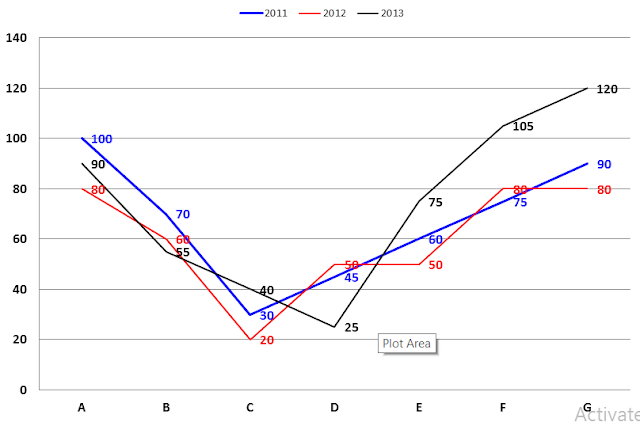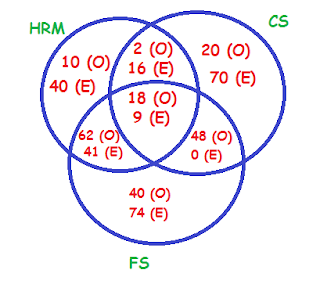## Data Interpretation for Bank Exams: Syndicate Bank, SBI PO

### Data Interpretation for Bank Exams: Syndicate Bank, SBI PO

Directions (1 – 5): Study the following graph carefully and answer accordingly:
The following graph shows the production of rice (in million tonnes) in seven states from 2011 to 2013.1. Which of the following states show the maximum percentage increase in the production of rice from 2011 to 2013?
a) C
b) G
c) E
d) A
e) None of these
2. By what per cent the production of rice in 2011 produced by state B is less than that by state F in 2013?
a) 50%
b) 33 1/3 %
c) 40%
d) 25%
e) None of these
3. Find the average production of rice (in million tonnes) by all states in 2012.
a) 55.42
b) 65
c) 60
d) 50.82
e) 70.67
4. The rice produced by states D and F together in 2011 is what per cent more than that by state B and D together in 2013?
a) 50%
b) 33.33%
c) 40%
d) 60%
e) None of these
5. Which of the following states shows a continuous decrease in the production of rice over the years?
a) A
b) B
c) C
d) G
e) None of these
Directions (6 – 10) Study the following graph carefully and answer the questions given below it:6. In which year is the value of sugar exported per tonne the highest?
a) 2009
b) 2008
c) 2010
d) 2012
e) 2013
7. In which of the following years is the percentage increase in the value of sugar exported over the previous year the minimum?
a) 2009
b) 2011
c) 2012
d) 2013
e) 2010
8. In how many years the quantity of sugar exported is more than the average quantity of sugar exported for the given period?
a) 1
b) 2
c) 3
d) 4
e) 5
9. What is the difference in the value of sugar per kg exported in 2009 and that in 2012?
a) Rs 2
b) Rs 1.50
c) Rs 1
d) Rs 5
e) Rs 2.50
10. From 2013 to 2014, if there is a 2.5% increase in the quantity of sugar exported and 40% increase in the value, then what is the value of sugar exported per kg in 2014?
a) Rs 11
b) Rs 12
c) Rs 13
d) Rs 14
e) Rs 15
Directions (11 – 15): Study the following information carefully to answer the given questions.
A chartered accountancy firm having 450 employees has sent all its employees for training in one or more areas among HRM, Computer skills and Financial skills. The employees are classified into two categories – Officers and Executives – in the ratio of 4 : 5. 10% of the Officers take training only in Computer skills. 16% of the Executives take training only in HRM, which is equal to the number of Officers taking training only in Financial skills. And 40% of the Officers are taking training in HRM and Financial skills both. 6% of the total employees are taking training in all the three areas and of these, two-third are Officers. 10% of the total employees are taking training in HRM and Computer skills both. The number of Officers taking training only in HRM is 25% of the number of Executives taking training only in HRM. 20% of the total number of employees are taking training only in Computer skills. The number of Executives taking training in HRM and Financial skills both is 20% of the total number of Executives. There is no such executive as takes training in both Financial skills and Computer skills but not in HRM. Among the employees who take training in both HRM and Computer skills but not in Financial skills, the ratio of officers to executives is 1 : 8
11. How many Executives are taking training in Financial skills?
a) 124
b) 120
c) Can’t be determined
d) 80
e) None of the above
12. How many Officers are taking training in Computer skills?
a) 22
b) 88
c) 58
d) 40
e) None of the above
13. How many Executives are taking training in HRM but not in Computer skills?
a) 90
b) 106
c) 81
d) 80
e) None of the above
14. What percent of the total number of Officers are taking training in Financial skills but not in HRM?
a) 70%
b) 44%
c) 40%
d) 35%
e) None of the above
15. How many employees are taking training in Financial skills?
a) 280
b) 295
c) 292
d) 287
e) None of the above
1. E)   2. B)   3. C   4. A)   5. B)    6. C)   7. A)   8. B)   9. B)   10. D)   11. )   12. )   13. )   14. )   15. )
1. E) There is maximum gap between 2011 and 2013 for state F. And maximum percentage increase is also for state F.
2. B) Required less % = 105 – 70 / 105 = 33 1/3 %
3. C) Avg. production = 80 + 60 + 20 + 50 + 50 + 80 + 80 / 7 = 60 million tonnes
4. A) Required more % = (45 + 75 – 55 + 25) / (55 + 25) × 100 = 50%
5. B) Given data shows that B state shows a continuous decrease in the production of rice over the years.
6. C) Value per tonne = Value/tone
7. A) % increase = (difference/Previous year) × 100
Now 2009 to 2010 = [(165 - 144)/144] × 100
8. B) Average quantity of export of suga = (12 + 15 + 10 + 13 + 20 + 22)/6 = 92/6, = 15.33 tonne
Thus, in the year 2012 and 2013, the export quantity of sugar was more than average quantity of sugar export.
9. B) Difference = [(230×1000)/ (20 × 1000)] - [(165×1000)/ (15 × 1000)] = 11.5 – 11, = Rs. 1.5
10. D) Quantity of sugar exported in 2014 = 1.25 × 22 tonnes = 27.5 tonnes
Value of sugar exported in 2014 = 1.4 × 275 = Rs. 385 thousand
Therefore value per kg in 2014 = [(1.4×275)/ (1.25 × 22)] = Rs. 14
(11 – 15):
Number of Officers = 450 × 4/9 = 200
Number of Executives = 450 × 5/9 = 25011. A) No. of Executives taking training in Financial skill = 74 + 41 + 9 = 124
12. B) Total no. of Officers taking training in Computer skills = 20 + 2 + 18 + 48 = 88
13. C) Number of Executives = 40 + 41 = 81
14. B) Required percentage = (40 + 48)/200 × 100 = 44%
15. C) Total no. of employees taking training in Financial skills = 62 + 41 + 18 + 9 + 48 + 0 + 40 + 74  = 292